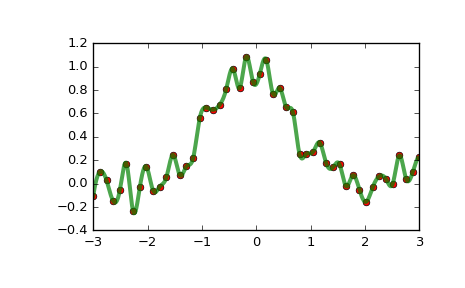# scipy.interpolate.InterpolatedUnivariateSpline¶

class scipy.interpolate.InterpolatedUnivariateSpline(x, y, w=None, bbox=[None, None], k=3, ext=0, check_finite=False)[source]

One-dimensional interpolating spline for a given set of data points.

Fits a spline y = spl(x) of degree k to the provided x, y data. Spline function passes through all provided points. Equivalent to UnivariateSpline with s=0.

Parameters: x : (N,) array_like Input dimension of data points – must be increasing y : (N,) array_like input dimension of data points w : (N,) array_like, optional Weights for spline fitting. Must be positive. If None (default), weights are all equal. bbox : (2,) array_like, optional 2-sequence specifying the boundary of the approximation interval. If None (default), bbox=[x, x[-1]]. k : int, optional Degree of the smoothing spline. Must be 1 <= k <= 5. ext : int or str, optional Controls the extrapolation mode for elements not in the interval defined by the knot sequence. if ext=0 or ‘extrapolate’, return the extrapolated value. if ext=1 or ‘zeros’, return 0 if ext=2 or ‘raise’, raise a ValueError if ext=3 of ‘const’, return the boundary value. The default value is 0. check_finite : bool, optional Whether to check that the input arrays contain only finite numbers. Disabling may give a performance gain, but may result in problems (crashes, non-termination or non-sensical results) if the inputs do contain infinities or NaNs. Default is False.

UnivariateSpline
Superclass – allows knots to be selected by a smoothing condition
LSQUnivariateSpline
spline for which knots are user-selected
splrep
An older, non object-oriented wrapping of FITPACK
BivariateSpline
A similar class for two-dimensional spline interpolation

Notes

The number of data points must be larger than the spline degree k.

Examples

>>> import matplotlib.pyplot as plt
>>> from scipy.interpolate import InterpolatedUnivariateSpline
>>> x = np.linspace(-3, 3, 50)
>>> y = np.exp(-x**2) + 0.1 * np.random.randn(50)
>>> spl = InterpolatedUnivariateSpline(x, y)
>>> plt.plot(x, y, 'ro', ms=5)
>>> xs = np.linspace(-3, 3, 1000)
>>> plt.plot(xs, spl(xs), 'g', lw=3, alpha=0.7)
>>> plt.show()Notice that the spl(x) interpolates y:

>>> spl.get_residual()
0.0


Methods

 __call__(x[, nu, ext]) Evaluate spline (or its nu-th derivative) at positions x. antiderivative([n]) Construct a new spline representing the antiderivative of this spline. derivative([n]) Construct a new spline representing the derivative of this spline. derivatives(x) Return all derivatives of the spline at the point x. get_coeffs() Return spline coefficients. get_knots() Return positions of interior knots of the spline. get_residual() Return weighted sum of squared residuals of the spline approximation. integral(a, b) Return definite integral of the spline between two given points. roots() Return the zeros of the spline. set_smoothing_factor(s) Continue spline computation with the given smoothing factor s and with the knots found at the last call.